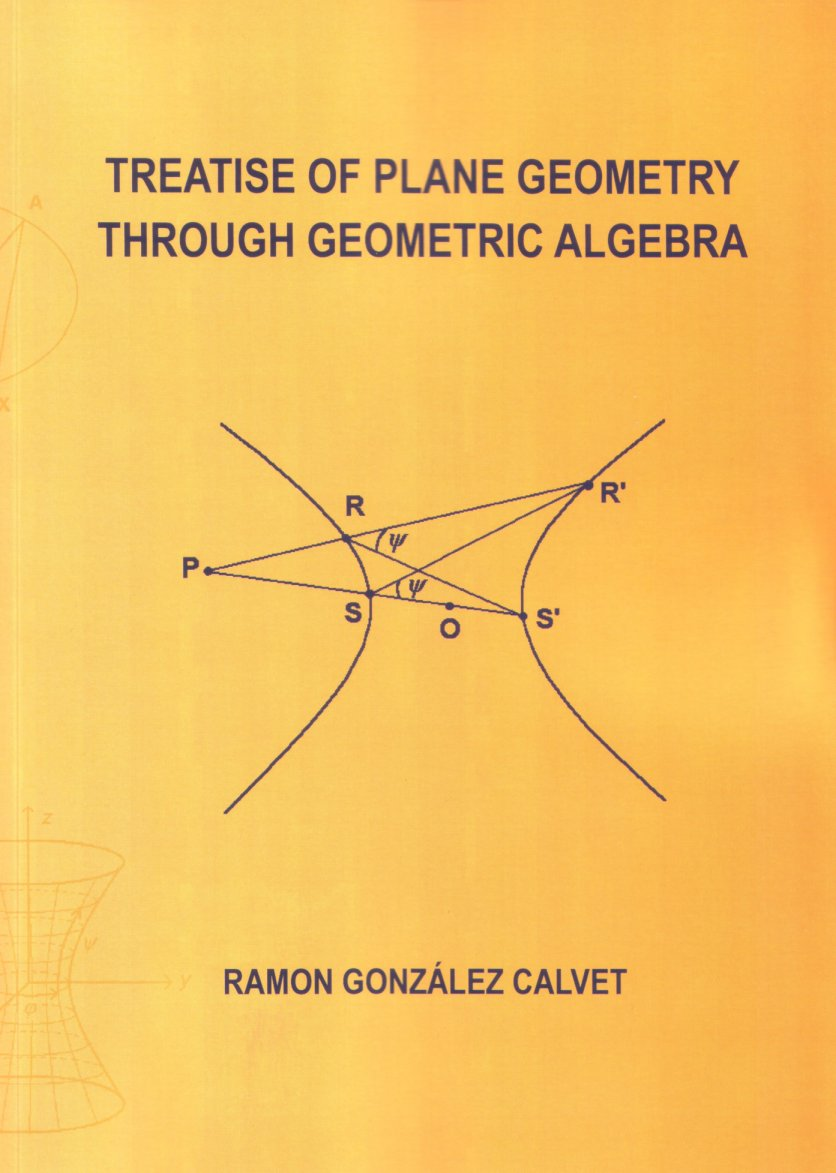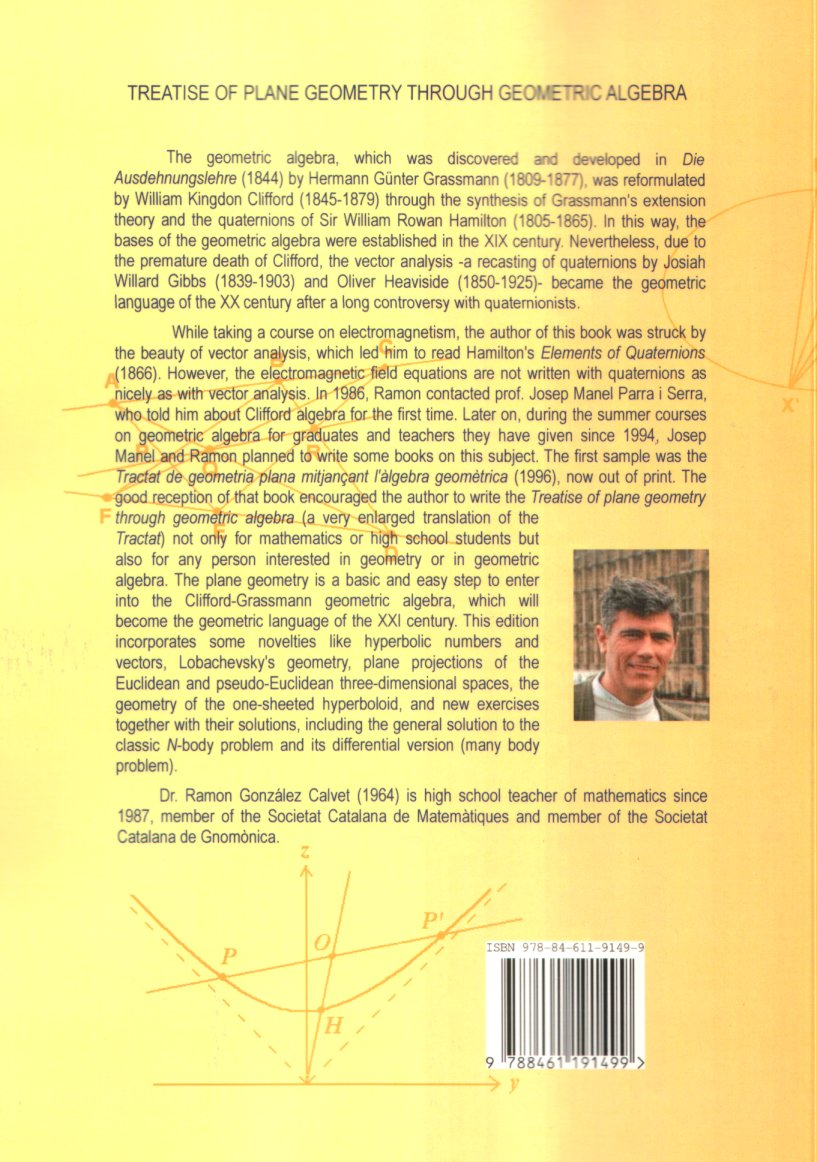TREATISE OF PLANE GEOMETRY THROUGH GEOMETRIC ALGEBRA               First printed edition  (November 2007)     306 pages with  143 figures and 123 solved problems. Paperback with size 20 x 28 cm. Price: 67,24 © Ramon González Calvet         ISBN: 978-84-611-9149-9The Treatise of plane geometry through geometric algebra is a very enlarged translation of the Tractat de geometria plana mitjançant l'àlgebra geomètrica. into English. Here the Clifford-Grassman Geometric Algebra is applied to solve geometric equations, which are like the algebraic equations but containing geometric (vector) unknowns instead of real quantities. The unique way to solve these kind of equations is by using an associative algebra of vectors, the Clifford-Grassmann Geometric Algebra. Using the CGGA we have the freedom of transposition and isolation of any geometric unknown in a geometric equation. Then the typical problems and theorems of geometry, which have been hardly proved till now by means of synthetic methods, are more easily solved through the CGGA. On the other hand, the formulas obtained from the CGGA can immediately be written in Cartesian coordinates, giving a very useful service for programming computer applications, as in the case of the notable points of a triangle.     This edition is a revision of the first electronic edition that incorporates the following novelties:     1) The geometry and trigonometry of the one-sheeted hyperboloid in the 3D-pseduoEuclidean space.     2) More exercises and their solutions including the general solution to the N-body problem and its differential version (many body problem).Updated May 13th, 2017

CONTENTS

First Part: The Euclidean vector plane and complex numbers

1. Euclidean vectors and their operations
Vector addition, 1.- Product of a vector and a real number, 2.- Product of two vectors, 2.- Product of three vectors: associative property, 5. Product of four vectors, 7.- Inverse and quotient of two vectors, 7.- Priority of algebraic operations, 8.- Geometric algebra of the vector plane, 9.- Exercises, 9.

2. A vector basis for the Euclidean plane
Linear combination of two vectors, 10.- Basis and components, 10.- Orthonormal bases, 11.- Applications of formulae for products, 11.- Exercises, 12.

3. Complex numbers
The subalgebra of complex numbers, 13.- Binomial, polar and trigonometric form of a complex number, 13.- Algebraic operations with complex numbers, 14.- Permutation of complex numbers and vectors, 17.- The complex plane, 18.- Complex analytic functions, 19.- Fundamental theorem of algebra, 24.- Exercises, 26.

4. Transformations of vectors
Rotations, 27.- Axial symmetries, 28.- Inversions, 29.- Dilations, 30.- Exercises, 30

Second Part: Geometry of the Euclidean plane

5. Points and straight lines
Translations, 31.- Coordinate systems, 31.- Barycentric coordinates, 33.- Distance between two points and area, 33.- Condition of collinearity of three points, 35.- Cartesian coordinates, 36.- Vectorial and parametric equations of a line, 36.- Algebraic equation and distance from a point to a line, 37.- Slope and intercept equations of a line, 40.- Polar equation of a line, 41.- Intersection of two lines and pencil of lines, 41.- Dual coordinates, 43.- Desarguess theorem, 48.- Exercises, 50.

6. Angles and elemental trigonometry
Sum of the angles of a polygon, 53.- Definition of trigonometric functions and fundamental identities, 54.- Angle inscribed in a circle and double-angle identities, 55.- Addition of vectors and sum of trigonometric functions, 56.- Product of vectors and addition identities, 57.- Rotations and de Moivre's identity, 58.- Inverse trigonometric functions, 59.- Exercises, 60.

7. Similarities and simple ratio
Direct similarity (similitude), 61.- Opposite similarity, 62.- Menelaus theorem, 63.- Cevas theorem, 64.- Homothety and simple ratio, 65.- Exercises, 67.

8. Properties of triangles
Area of a triangle, 68.- Medians and centroid, 69.- Perpendicular bisectors and circumcentre, 70.- Angle bisectors and incentre, 72.- Altitudes and orthocentre, 73.- Euler's line, 76.- Fermat's theorem, 77.- Exercises, 78.

9. Circles
Algebraic and Cartesian equations, 80.- Intersections of a line with a circle, 80.- Power of a point with respect to a circle, 82.- Polar equation, 82.- Inversion with respect to a circle, 83.- The nine-point circle, 85.- Cyclic and circumscribed quadrilaterals, 87.- Angle between circles, 89.- Radical axis of two circles, 89.- Exercises, 91.

10. Cross ratios and related transformations
Complex cross ratio, 92.- Harmonic characteristic and ranges, 94.- Homography (Möbius transformation), 96.- Projective cross ratio, 99.- Points at infinity and homogeneous coordinates, 102.- Perspectivity and projectivity, 103.- Projectivity as a tool for theorem demonstrations, 108.- Homology, 110.- Exercises, 115.

11. Conics
Conic sections, 117.- Two foci and two directrices, 120.- Vectorial equation, 121.- Chasles' theorem, 122.- Tangent and perpendicular to a conic, 124.- Central equations for ellipse and hyperbola, 126.- Diameters and Apollonius' theorem, 128.- Conic passing through five points, 131.- Pencil of conics passing through four points, 133.- Conic equation in barycentric coordinates and dual conic, 133.- Polarities, 135.- Reduction of the conic matrix to diagonal form, 136.- Exercises, 137.

Third part: Pseudo-Euclidean geometry

12. Matrix representation and hyperbolic numbers
Rotations and the representation of complex numbers, 139.- The subalgebra of hyperbolic numbers, 140.- Hyperbolic trigonometry, 141.- Hyperbolic exponential and logarithm, 143.- Polar form, powers and roots of hyperbolic numbers, 144.- Hyperbolic analytic functions, 147.- Analyticity and square of convergence of power series, 150.- About the isomorphism of Clifford algebras, 152.- Exercises, 153.

13. The hyperbolic or pseudo-Euclidean plane
Hyperbolic vectors, 154.- Inner and outer products of hyperbolic vectors, 155.- Angles between hyperbolic vectors, 156.- Congruence of segments and angles, 158.- Isometries, 158.- Theorems about angles, 160.- Distance between points, 160.- Area in the hyperbolic plane, 161.- Diameters of the hyperbola and Apollonius theorem, 163.- The law of sines and cosines, 164.- Hyperbolic similarity, 167.- Power of a point with respect to a hyperbola with constant radius, 168.- Exercises, 169.

Fourth part: Plane projections of three-dimensional spaces

14. Spherical geometry in the Euclidean space
The geometric algebra of the Euclidean space, 170.- Spherical trigonometry, 172.- The dual spherical triangle of a given triangle, 175.- Right spherical triangles and Napiers rule, 176.- Area of a spherical triangle, 176.- Properties of the projections of the spherical surface, 177.- Central or gnomonic projection, 177.- Stereographic projection, 180.- Orthographic projection, 181.- Lamberts azimuthal equivalent projection, 182.- Spherical coordinates and cylindrical equidistant (plate carré) projection, 183.- Mercator projection, 184.- Cylindrical equivalent projection, 184.- Conic projections, 185.- Exercises, 186.

15. Hyperboloidal geometry in the pseudo-Euclidean space
The geometric algebra of the pseudo-Euclidean space, 189.- The hyperboloid of two sheets (Lobachevskian surface), 191.- Central projection (Beltrami disk), 192.- Lobachevskian trigonometry, 197.- Stereographic projection (Poincaré disk), 199.- Azimuthal equivalent projection, 201.- Weierstrass coordinates and cylindrical equidistant projection, 202.- Cylindrical conformal projection, 203.- Cylindrical equivalent projection, 204.- Conic projections, 204.- About the congruence of geodesic triangles, 206.- The hyperboloid of one sheet, 206.- Central projection and arc length on the one-sheeted hyperboloid, 207.- Cylindrical projections, 208.- Cylindrical central projection, 209.- Cylindrical equidistant projection, 210.- Cylindrical equivalent projection, 210.- Cylindrical conformal projection, 210.- Area of a triangle on the one-sheeted hyperboloid, 211.- Trigonometry of right triangles, 214.- Hyperboloidal trigonometry, 215.- Dual triangles, 218.- Summary, 221.- Comment about the names of the non-Euclidean geometry, 222.- Exercises, 222.

16. Solutions to the proposed exercises
1.
Euclidean vectors and their operations, 224.- 2. A vector basis for the Euclidean plane, 225.- 3. Complex numbers, 227.- 4. Transformations of vectors, 230.- 5. Points and straight lines, 231.- 6. Angles and elemental trigonometry, 241.- 7. Similarities and simple ratio, 244.- 8. Properties of triangles, 246.- 9. Circles, 257.- 10. Cross ratios and related transformations, 262.- 11. Conics, 266.- 12. Matrix representation and hyperbolic numbers, 273.- 13. The hyperbolic or pseudo-Euclidean plane, 275.- 14. Spherical geometry in the Euclidean space, 278.- 15. Hyperboloidal geometry in the pseudo-Euclidean space, 284.

Bibliography, 293.

Internet bibliography, 296.

Index, 298.

Chronology of the geometric algebra, 305.

 Come back